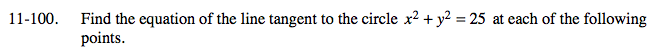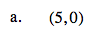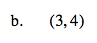### Home > A2C > Chapter 11 > Lesson 11.2.5 > Problem11-100

11-100.Find the equation of the line from the center of the circle to this point.

y = 0

Now find the equation of the line that is perpendicular to this line that passes through this point.

x = 5See part (a) for the method.

$y=-\frac{3}{4}x+\frac{25}{4}$